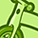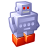Announcements
MAINTENANCE ALERT: Dec. 12th starting 9 AM CET. Community will be read-only. GET DETAILS
cancel
Showing results for
Did you mean:Creator II

Hi

I have this script and give an error, did you know why?

LET vExecTime=UTC();

Set vLastExecTime=0;

if (not IsNull(QvdCreateTime('\$(VStore)INVENTTRANS_INCREMENTAL.qvd'))

,

From \$(VStore)INVENTTRANS_INCREMENTAL.qvd(qvd);

);

Many thank's

1 Solution

Accepted SolutionsSpecialist III

yes the "if" and "then" have to be in the same line

6 RepliesSpecialist III

use an if ... then instead of if ()

# If..then..elseif..else..end if

The if..then control statement is a script selection construct forcing the script execution to follow different paths depending on one or several logical conditions. The syntax is:

if condition then

[ statements ]

{ elseif condition then

[ statements ] }

[ else

[ statements ] ]

end if

Where:

condition is a logical expression which can be evaluated as true or false.

statements is any group of one or more QlikView script statements.

Since the if..then statement is a control statement and as such is ended with either a semicolon or end-of-line, each of its four possible clauses (if..then, elseif..then, else and end if) must not cross a line boundary.

Examples:

if a=1 then

sql select e, f, g from tab1;

end if

if a=1 then; drop table xyz; end if;

if x>0 then

elseif x<0 then

else

end ifCreator II
Author

Hi Ramon,

Many thank's but still with problems 😞

see the picture

EduardMVP

Hi Eduard, following Ramon instructions:

Since the if..then statement is a control statement and as such is ended with either a semicolon or end-of-line, each of its four possible clauses (if..then, elseif..then, else and end if) must not cross a line boundary.Specialist III

yes the "if" and "then" have to be in the same lineCreator II
Author

Hi Ruben, But i did not understand 😞Creator II
Author

Many many thank's Ramon!!!!Community Browser